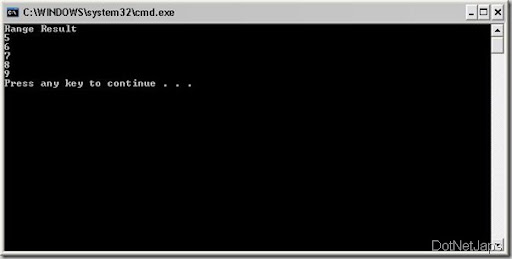Wednesday, June 23, 2010

## Range Operator in Linq.

Linq is almost providing all the functionalities and i have found one another great operator called range operator which will return a sequence of integer number from start point to number of count. Here is the signature of range operator in Linq.
`public static IEnumerable<int> Range(int start, int count)`
Here the Start means the starting integer of the sequence and count means the number of sequence you want from starting integer. Let’s take a simple example for that where will print 5 to 9 sequence with the help of range operator. Here is the code for that.
```using System;
using System.Collections.Generic;
using System.Linq;
using System.Text;

namespace ConsoleApplication1
{
class Program
{
static void Main(string[] args)
{
var RangeResult = Enumerable.Range(5, 5);
Console.WriteLine("Range Result");
foreach (int num in RangeResult)
{
Console.WriteLine(num);
}
}

}
}```
And here is the output for that as expected.Technorati Tags: ,,Share: